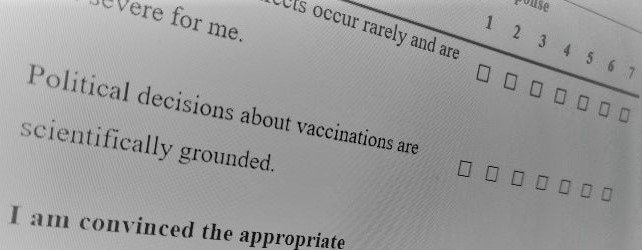Measure & MaterialsSupplementary Material

We provide supplementary material on OSF. There you can find further information about analyses and data used in our studies.

Scoring Information

All items should be scored so that high values indicate high vaccination readiness. Therefore, the following items should be recoded for data analysis as following:

# Complacency
df$cmpcy_01 <- recode(df$cmpcy_01, '1' = 7, '2' = 6,'3' = 5, '4'= 4, '5'= 3, '6' = 2, '7' = 1)
df$cmpcy_02 <- recode(df$cmpcy_02, '1' = 7, '2' = 6,'3' = 5, '4'= 4, '5'= 3, '6' = 2, '7' = 1)

# Constraints
df$const_03 <- recode(df$const_03, '1' = 7, '2' = 6,'3' = 5, '4'= 4, '5'= 3, '6' = 2, '7' = 1)

# Calcualation
df$calc_01 <- recode(df$calc_01, '1' = 7, '2' = 6,'3' = 5, '4'= 4, '5'= 3, '6' = 2, '7' = 1)
df$calc_02 <- recode(df$calc_02, '1' = 7, '2' = 6,'3' = 5, '4'= 4, '5'= 3, '6' = 2, '7' = 1)
df$calc_03 <- recode(df$calc_03, '1' = 7, '2' = 6,'3' = 5, '4'= 4, '5'= 3, '6' = 2, '7' = 1)

# Conspiracy
df$consp_01 <- recode(df$consp_01, '1' = 7, '2' = 6,'3' = 5, '4'= 4, '5'= 3, '6' = 2, '7' = 1)
df$consp_02 <- recode(df$consp_02, '1' = 7, '2' = 6,'3' = 5, '4'= 4, '5'= 3, '6' = 2, '7' = 1)
df$consp_03 <- recode(df$consp_03, '1' = 7, '2' = 6,'3' = 5, '4'= 4, '5'= 3, '6' = 2, '7' = 1)